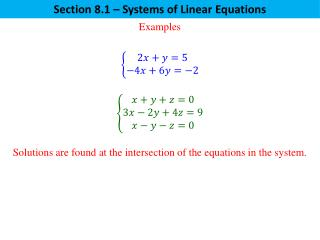# Examples - PowerPoint PPT PresentationDownload PresentationExamples

Examples
Download Presentation## Examples

- - - - - - - - - - - - - - - - - - - - - - - - - - - E N D - - - - - - - - - - - - - - - - - - - - - - - - - - -
##### Presentation Transcript

1. Section 8.1 – Systems of Linear Equations Examples Solutions are found at the intersection of the equations in the system.

2. Section 8.1 – Systems of Linear Equations Types of Solutions Consistent System Consistent System Inconsistent System One solution Infinite solutions No solution

3. Section 8.2 – Systems of Linear Equations - Matrices Using Matrices to Solve Systems of Equations Matrix – a rectangular array of numbers Identifying the Entries in a Matrix Entries are lower case letters with subscripts . The first subscript refers to the row of the entry. The second subscript refers to the column of the entry. Examples This refers to the entry in the 2nd row, 1st column.

4. Section 8.2 – Systems of Linear Equations - Matrices Using Matrices to Solve Systems of Equations Augmented Matrix – a matrix that is used to solve a system of equations. Augmented matrix Augmented matrix

5. Section 8.2 – Systems of Linear Equations - Matrices Using Matrices to Solve Systems of Equations Given the augmented matrix, write the system of equations. System of Equations System of Equations

6. Section 8.2 – Systems of Linear Equations - Matrices Using Matrices to Solve Systems of Equations The use of Elementary Row Operations is required when solving a system of equations using matrices. Elementary Row Operations I. Interchange two rows. II. Multiply one row by a nonzero number. III. Add a multiple of one row to a different row.

7. Section 8.2 – Systems of Linear Equations - Matrices Using Matrices to Solve Systems of Equations The solution to the system of equations is complete when the augmented matrix is in Row Echelon Form. Row Echelon Form A matrix is in row echelon form (ref) when it satisfies the following conditions. • The first non-zero element in each row, called the leading entry, is 1. Each leading entry is in a column to the right of the leading entry in the previous row. Rows with all zero elements, if any, are below rows having a non-zero element.

8. Section 8.2 – Systems of Linear Equations - Matrices Using Matrices to Solve Systems of Equations Reduced Row Echelon Form A matrix is in reduced row echelon form (rref) when it satisfies the following conditions. • The matrix is in row echelon form (i.e., it satisfies the three conditions listed for row echelon form. The leading entry in each row is the only non-zero entry in its column.

9. Section 8.2 – Systems of Linear Equations - Matrices Use matrices to solve the following systems of equations. (pg. 569 #58)

10. Section 8.2 – Systems of Linear Equations - Matrices Use matrices to solve the following systems of equations.

11. Section 8.2 – Systems of Linear Equations - Matrices Consistent or Inconsistent System?

12. Section 8.2 – Systems of Linear Equations - Matrices Use matrices to solve the following systems of equations. (pg. 569 #66)